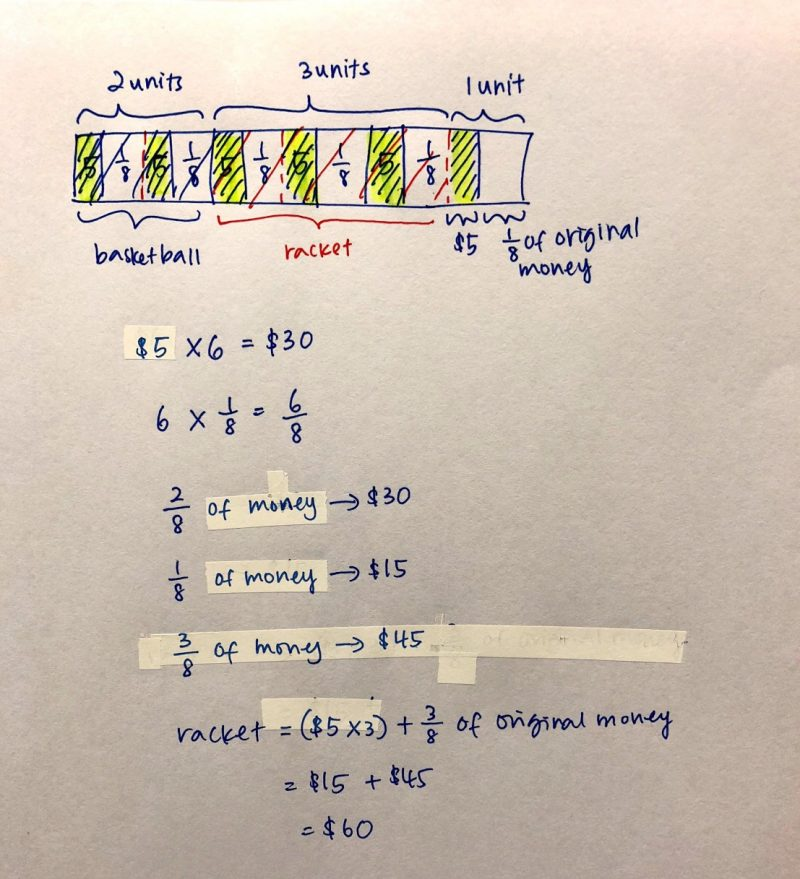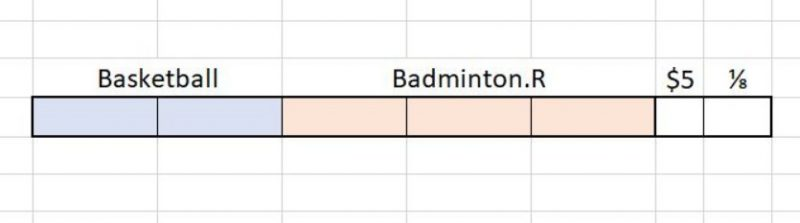# Question

This is the way I would explain to my kids.

a) 1/3 spent on basketball, therefore remaining amount of money is 2/3

b) 3/4 of 2/3 spent on badminton racquet, therefore 3/4 x 2/3 = 6/12 (or 1/2)

c) 1/3 + 1/2 of the money spent on the above, therefore remaining amount is 1 – (1/3 + 1/2) = 1/6

d) 1/6 = \$5 + 1/8. If we shift the equation around, 1/6 – 1/8 = \$5, therefore 1/24 = \$5

e) Half the initial amount of money was spent on the badminton racquet, therefore 12 x \$5 = \$60

So the cost of the badminton racquet is \$60.

0 Replies 1 Like ✔Accepted Answer0 Replies 0 LikesSorry. I don’t know how to explain clearly, but the answer \$60 is correct.

a) 1U = 1/8 + 5

b) 5U + 5 = 7/8

5U = 7/8 – 5

c) To get rid of the fraction, (a) times 7

7U = (1/8 + 5) * 7

= 7/8 + 35

d) Using (c) – (b):

7U – 5U = (7/8 + 35) – (7/8 – 5)

2U = 40 (*note: 35 minus, minus 5 = 35+5)

3U = (40/2) * 3

= \$60

0 Replies 1 Like